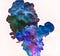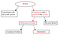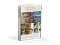Photo by rawpixel on Unsplash

All models are wrong but some are useful — George Box

Real numbers are not real. The argument is simple, real numbers cannot reflect reality (i.e. not real) because they assume to have infinite precision. Infinite precision is an impossibility in nature because it assumes that an infinite amount of information is contained in a single real number. Therefore, we must assume that reality uses numbers with finite precision. A real number is only significant after a certain number right of the decimal point.

in most numerical simulations infinite precision is not an immediate concern. That’s because finite precision numbers have always been used to approximate infinite precision real numbers. Computationally, the only time you really need extremely high precision numbers is in cryptography. However, a majority of numerical simulation code requires just 64-bit floating point numbers.

The question that however needs to be asked is, how does this relate to existing analytic theories of reality? Newton’s laws and Maxwell equations, both classical physics theories, are analytic tools (i.e. calculus) that assume infinite precision numbers (i.e. real numbers). What are the consequences if we assume finite precision numbers? This idea is explored in a recent paper by Nicolas Gisin (Experimental and theoretical physicist at the University of Geneva). In his paper “Indeterminism in Physics, Classical Chaos and Bohmian Mechanics. Are Real Numbers Really Real?” he emphasizes:

Mathematical real numbers are physical random numbers.

The origins of classical chaos originate from the finite precision of quantities that describe nature. This implies that the determinism of classical physics is not real. To assume determinism is tantamount to assuming a single number contains infinite information, an obvious absurdity. The implication of this is that all our analytic equations of reality (the models that we have symbolic closed forms) are all approximations of reality and NOT the reverse as commonly assumed. Mathematics assumes infinite precision so as to derive new knowledge, but this is all based on an approximation. Infinite precision employs a deterministic abstraction that demands that absolute knowledge is available when in reality it is not. I purposely used the word ‘approximation’ instead of ‘abstraction’ to convey the fact that infinite precision overshoots the precision intrinsic in reality. A more prevalent myth is that numerical methods undershoot reality due to its lack of precision. It is the delusion of users of mathematics to believe that analytic equations are the ultimate truth when in truth the real physics demands indeterminism (BTW, see also the Rationalist Delusion). In the end, all we have are abstract models of reality, finite or infinite-precision models that should be treated in equal standing.

I’ve written previously about the issues with assuming the existence of infinity as it relates to expressing randomness. There are other assumptions in conventional mathematics that are equally problematic in representing reality. The other one is the assumption of the excluded middle. This is found in proofs of contradiction and the double negative. There is an alternative kind of logic known as intuitionistic logic that demands only what can be constructed is what can be proven. In fact, one can argue that Gödel’s incompleteness theorem is proof that invalidates classical mathematics.

How does this all relate then to the field of Deep Learning? Here’s where I see the problem, a majority of the papers conjure up formal arguments based on mathematics that assume the existence of infinity, infinite precision or the excluded middle. These are all nice to have included having a convincing argument about the validity of a method. Unfortunately, these arguments are all based on concepts that aren’t even real. All of these mathematical tools are crutches to our true understanding of reality. Closed analytic solutions are nice, but they are applicable only for simple configurations of reality. At best, they are toy models of simple systems. Physicists have known for centuries that the three-body problem or three dimensional Navier Stokes do not afford a closed form analytic solutions. This is why all calculations about the movement of planets in our solar system or turbulence in a fluid are all performed by numerical methods using computers.

This notion that a formal proof using real analysis is true, is a delusion. It will always be an approximation of the truth. The proof of something that works is in the actual simulation. You have to run the computation to prove that something is actually true. Deep Learning works despite the optimization theorist proclaiming that high-dimensional spaces are non-convex and thus require exponential time to converge. Deep Learning works because the theory is an approximation, and can only be validated through experimental work. Therefore, never be discouraged by analytic proofs that a method is an impossibility.

The motivation for this discussion revolves around the idea of numerical methods for simulations versus black box Deep Learning generative models. Numerical Analysis the bread and butter of Computational Science (i.e. Scientific Computing) are derived directly from formulated mathematical models:https://en.wikipedia.org/wiki/Computational_science

There are numerous algorithms and methods in computational science that are used to approximate and stabilize the simulation of mathematical models. The pragmatic question is can these numerical simulations be performed in a more computationally efficient way using DL generative models?

One very strange departure of DL models is that, despite being formulated through continuous mathematics, computations do not require high precision. It is typical to find training using 16-bit floating point numerics and inference requiring even less precision (i.e. 8 bit). This is in stark contrast with HPC simulations that required higher precision 64bit floating point. This is a tell on the fundamental difference between the two approaches. Perhaps generative models require less precision that descriptive models. Why do bottom-up generative models require less numeric precision than top-down numerical models? In fact, if you compare the computational requirements of a top-down Monte Carlo simulation, the computational requirements are tremendous relative to a DL model. This is why Probabilistic Graph Models don’t scale like DL models.

I suspect traditional numerical methods are appealing due to the illusion of control they provide. Can we build DL methods that afford an equivalent richness of controls? How can we develop DL models with the same kind of confidence as computational science models? DL generative models for fluid and smoke have previously successfully be demonstrated:https://cims.nyu.edu/~schlacht/CNNFluids.htm

The problem, however, is that (despite this looking like the real thing for humans) does this actually approximate the true physics?

The justification for a DL generative approach is precise because the mathematical models where numerical methods are derived are approximations, to begin with, and thus also do not necessarily exhaustively represent the physics. The main argument against DL models is that they don’t represent any physics, although they seem to generate simulations that do look realistically like physics. However, you can train a DL model to mirror the behavior of a simulation. This would imply that it would be an approximation approximating an approximation (simulation) that approximates another approximation (mathematical model).

The difference is that DL models potentially bridge the gap with higher fidelity than the best simulation. This can be done because DL can potentially learn from aggregate simulation models. This is the approach that many in weather simulation employ. That is the use of an ensemble of models for prediction.

The potential for using DL generative models to replace traditional numerical methods is vast. Numerical methods are essential for many kinds of manufacturing of advanced materials and engines. Accurate simulations permit discovery of problems prior to expensive manufacturing, this not only drastically reduces cost but permits faster iteration in design. In fact, the coupling of faster DL generative solution with Reinforcement Learning algorithms can lead to a massive combinatorial search of new designs that could not have been feasibly discovered in real life.

What is needed now is an exploration into methodologies that are parallel to the methods in computational science. There is a wealth of knowledge that has accumulated over the years to bring stability and accuracy to the methods of computational science. These methods may by analogy be applied by DL generative methods. The difference is that rather than being handcrafted, these methods are grown.

Further ReadingExplore Deep Learning: Artificial Intuition: The Improbable Deep Learning Revolution

Written by

## Intuition Machine

#### Deep Learning Patterns, Methodology and Strategy

Welcome to a place where words matter. On Medium, smart voices and original ideas take center stage - with no ads in sight. Watch
Follow all the topics you care about, and we’ll deliver the best stories for you to your homepage and inbox. Explore
Get unlimited access to the best stories on Medium — and support writers while you’re at it. Just \$5/month. Upgrade